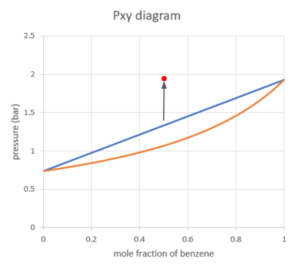# 31 Pxy Diagram

Learning Objectives

By the end of this section, you should be able to:

Read Pxy diagrams for binary mixtures

Find bubble points and dew points on a Pxy diagram

Calculate the mole fraction of substances in the vapour and liquid states

The following diagram is the Pxy diagram for a benzene-toluene mixture at a constant temperature of 373K. They y-coordinates show the pressure of the mixture and the x-coordinates show the mole fraction of benzene in liquid and/or vapour phases.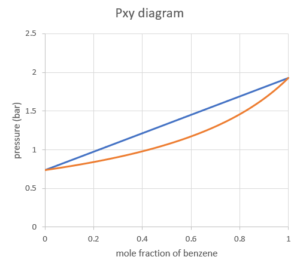Higher pressure forces molecules to come closer together, which causes the molecules to be more likely to condense from vapour to liquid. From top to bottom, the regions are liquid region, vapour-liquid equilibrium region, vapour region, which is the opposite from the Txy diagram: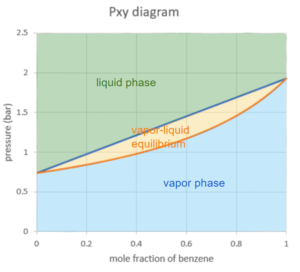Suppose we have a mixture with 0.5 mole fraction of benzene at a pressure of 0.5 bar, and we slowly increase the pressure while keeping the temperature constant at 373K.

We can locate our starting point using the pressure and mole fraction of benzene: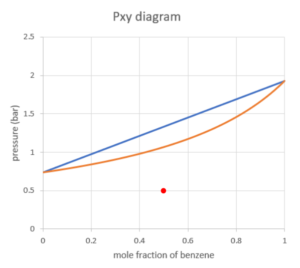The point is fully in the vapour region, which means the mixture of benzene and toluene is in the vapour phase at a pressure of 0.5 bar and temperature of 373K.

As we keep increasing the pressure, the point will hit the first curve on the boundary of the vapour region. This point is the dew point of the system. At the dew point, the first drop of liquid is being formed. The composition of the first liquid can be found using the x-coordinate of the point on the other curve where the pressure is the same.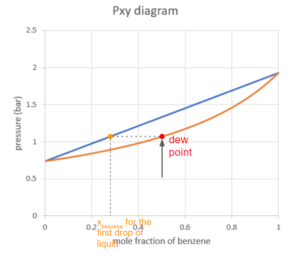If we keep increasing the pressure while keeping the temperature constant, the point will move up into the vapour-liquid equilibrium region. Similarly to the Txy diagram, we can read the mole fraction of benzene by drawing a horizontal line through the point and reading the x-coordinate where the line hits the two curves.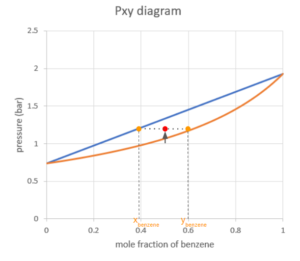As the pressure goes up, the system will hit the bubble point, where the last drop of vapour condenses into a liquid.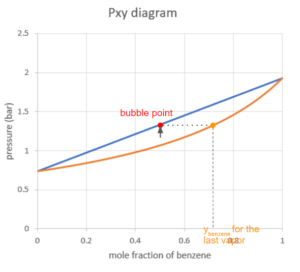Notice that the line connecting all the bubble points at different mole fractions appears to be linear. We can show why this occurs using Raoult’s law:

Starting with the total pressure in the system being the sum of individual partial pressures, we replace each partial pressure with the liquid mole fraction and vapour pressure (using Raoult’s law):
\begin{align*}
P=p_{1}+p_{2} & =x_{1}×p^*_{1}(T)+x_{2}×p^*_{2}(T)\\
& =x_{1}×p^*_{1}(T)+(1-x_{1})×p^*_{2}(T)\\
&= x_{1}×[p^*_{1}(T)-p^*_{2}(T)]+p^*_{2}(T)
\end{align*}

This equation is in a linear form, where $[p^*_{1}(T)-p^*_{2}(T)]$ is the slope and $p^*_{2}(T)$ is the y-intercept. Because the Pxy diagrams assume constant temperature, the vapour pressures are constant.

When we raise the pressure of the system over the dew point, the system will stay fully in the liquid region. There will be only liquid in the system and the composition of the liquid will be equal to the overall system composition.